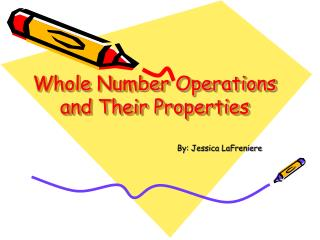# Whole Number Operations and Their Properties - PowerPoint PPT PresentationDownload PresentationWhole Number Operations and Their Properties

Whole Number Operations and Their PropertiesDownload Presentation## Whole Number Operations and Their Properties

- - - - - - - - - - - - - - - - - - - - - - - - - - - E N D - - - - - - - - - - - - - - - - - - - - - - - - - - -
##### Presentation Transcript

1. Whole Number Operations and Their Properties By: Jessica LaFreniere

2. This PowerPoint was designed to help students gain a better knowledge on whole number operations, and their properties. This PowerPoint will include definitions, and examples of each of the following: Commutative Property of Addition and Multiplication Associative Property Distributive Property The Zero Property of Addition The Zero Property of Multiplication The Multiplicative identity Order of Operations Below are links that will help answer any other questions you may have on this topic: Operations Lesson on Order of Operations Order of Operations (PEMDAS) Properties of Real Numbers Glossary of Properties

3. Commutative Property of Addition and Multiplication Addition and Multiplication are commutative: switching the order of two numbers being added or multiplied does not change the result. When adding numbers, it doesn't matter which number comes first, the sum will be the same. Another way to look at it is, buying two things in different order still will cost the same. Examples: 100 + 8 = 8 + 100 100 × 8 = 8 × 100

4. Associative Property Addition and multiplication are associative: the order that numbers are grouped in addition and multiplication does not affect the result. Examples: (2 + 10) + 6 = 2 + (10 + 6) = 18 2 × (10 × 6) = (2 × 10) × 6 =120

5. Distributive Property The Distributive Property is an algebra property which is used to multiply a single term and two or more terms inside a set of parentheses. Examples: 10 × (50 + 3) = (10 × 50) + (10 × 3) 3 × (12+99) = (3 × 12) + (3 × 99)

6. The Zero Property of Addition Adding 0 to a number leaves it unchanged. We call 0 the additive identity. Example: 88 + 0 = 88

7. The Zero Property of Multiplication Multiplying any number by 0 gives 0. Example: 88 × 0 = 0 0 × 1003 = 0

8. The Multiplicative Identity We call 1 the multiplicative identity. Multiplying any number by 1 leaves the number unchanged. Example: 88 × 1 = 88

9. Order of Operations The order of operations for complicated calculations is as follows: • Perform operations within parentheses. • Multiply and divide, whichever comes first, from left to right. • Add and subtract, whichever comes first, from left to right. Example: 1 + 20 × (6 + 2) ÷ 2 = 1 + 20 × 8 ÷ 2 = 1 + 160 ÷ 2 = 1 + 80 = 81.